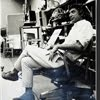## Eugene Ray SDSU 1970'sEugene Ray 1970's. photo credit: Tom Davis

## Friday, September 27, 2013

### THIS BLOG IS FOR UFO CYNICS & ATHIESTS

+OBTAIN "OPEN MINDS" MAGAZINE+
>(barnes and noble-bookstar etc)<
^^^^^^^^^^^^^^^^^^^
(>french and english UFO files<
>alone are profound but also<)
^^^^^^^^^^^^^^^^^^
+READ PAGES 50-55, THEN IF YOU+
>have any doubts you are
>beyond any hope for<
+ENLIGHTENMENT WHATEVER+
^^^^^^^^^^^^^^^^^^
>remember my sighting of 1947 made<
(+EYE WITNESS TO+
+THE COSMOS+)
>my life what it became and i want<
^^^^^^^^^^^^^^^^^^^^
+"NOT FROM THIS WORLD"+
>(marshall to roosevelt)<
+1942 USA CONCLUSION<
>(secret-mind boggling)<
^^^^^^^^^^^^^^^

+HONEST SCIENTIST LYTLE+
>top seer-inventor <
+REVEALS THE TRUTH+
>five "open minds" photos<
^^^^^^^^^^^^^^^^^^

+CURRENT ISSUE+
>issue22, october, november, 2013<
>(just out on the newsstands)<
^^^^^^^^^^^^^^^^^^

+ROSWELL UFO CRASH SECRETS+
>are the focus of our blog tonight<
^^^^^^^^^^^^^^^^^^^^^

+CHESTER LYTLE , (GENIUS)+
>left, inventor w/ 16 government patents<
>including the hydrofoil, codirector<
>of the u.s. manhattan project<
^^^^^^^^^^^^^^^^^
+DONALD SCHMITT+
>right, famed veteran UFO expert, coauthor<
>of several profound books on Roswell<
>codirector of j. allen hynek center<
^^^^^^^^^^^^^^^^^^^^^^^

+"LIFE ELSEWHERE IN SPACE"+
>scientist Chester Lytle open view<
>in this letter to donald schmitt<
^^^^^^^^^^^^^^^^^^^^

+EXTRATERRESTRIALS+
>confirmed in this profound letter by
>Chester Lytle former codirector of<
>the secret govt. manhattan project<
^^^^^^^^^^^^^^^^^^^^^^
+OVERVIEW+
>as these early scientists w/ knowledge of<
>the truth of the roswell crash approach<
>their death many have divulged the<
+ROSWELL REVELATION+
^^^^^^^^^^^^^^^^^^^^^
+"NOT OF THIS WORLD"+
^^^^^^^^^^^^^^^^^^^
>note : as i was writing this, a program<
>on "coast to coast" night radio has
>coverage of a huge space object<
+"SIMILAR TO THE SUN"+
^^^^^^^^^^^^^^^^^^

eugene ray, mfa, architect
professor emeritus, sdsu